RF SNR / SINAD / SINR   There are several similar but a little bit different terminology that indicates the ratio between the wanted signal and the unwanted noise. These terms are confusing almost everybody. I will try to explain the concept of these terms as much as practical sense (hopefully). In many case, it would be much easier to understand if you understand on what purpose (in what context) these are used.       SNR (Signal to Noise Ratio)   SNR stands for 'Signal to Noise Ratio'. It is pretty much self-explanatory and it would not need much explanation. It is just the ratio of Signal Power and Noise Power as described below in mathematical form.SNR can be represented in a graphical form as shown below.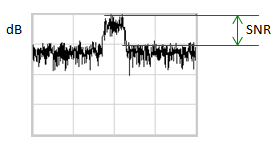SNR can be either positive and negative value if you represent it in dB scale. Negative SNR means that Signal power is lower than the noise power. You may think communication would be impossible in the negative SNR condition, but in reality there is communication system (technology) which is designed to work mostly in such a condition (e.g, CDMA, WCDMA).   Why SNR is important ? It is because SNR is one of the most important indicator to represent signal quality. You may think Signal Power is the most important factors for signal quality, but in theory Signal power alone does not mean anything in terms of representing signal quality which help you predict how much error will happen for your communication system. Even if your signal power is very strong, you would not get good communication result (low error or no error) if the noise power is high as well. On the contrary, even if the signal power is very low, you would get good communication result if the noise power is much lower than the signal power. This is why in most communication text book or in most of measurement process, SNR rather than the absolute signal power is used as evaluation/test criteria.   Now let's think of how to measure SNR. You can get the rough estimate of SNR for a certain signal using a spectrum analyzer, but it may not be as easy as it sound to measure the accurate SNR since ideally this measurement should be done at RBW of 1 Hz. However, if you have to measure SNR in a communication device (not in test equipment), you cannot use the same method as spectrum analyzer. In that case, the device use very complicated signal processing algorithm to estimate SNR and the method itself tend to be different depending on communication technology.   How does SNR impact the performance of a system (especially on the reciever of a transmission system) ? I think following plots would give you an intuitive understanding of this. As you see, as SNR decreases the quality of the signal gets poorer (higher noise level). As a result, Bit Error Rate (BER) will increase and Sensitity will decrease. (Note : Noise added to this example is AWGN. See AWGN page for the details of the relationship between SNR and AWGN)   In the following plots, the red dots indicate the ideal constellation with almost no error and the black dots represents the statistical location of each data points with noises. You can say, the farther a black dot is from the red dots, the higher probable errors (Bit Error) occur. In this example, you see three cases of QAM constellation and each case is exposed to error with different SNR. You would notice that as SNR goes lower the range of constellation spread goes wider. It means.. with the same modulation scheme .. as SNR goes lower, the probablity of error goes higher. If you are not familiar with this kind of concept, please give some more time until you understand the concept.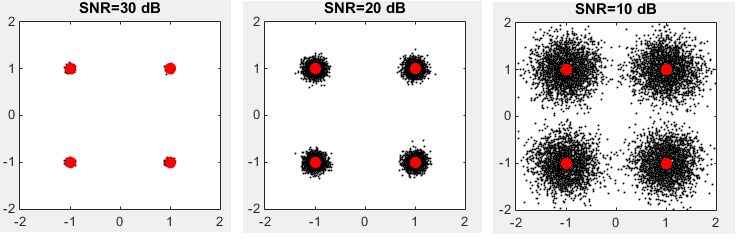Now let's describe on the relationship between SNR and Bit Error Rate in more quantitative way. If you had chance with articles or papers /thesis about communication technology (especially with anything related to transmitter, reciever technology), you would have seen the plots as shown at the bottom right.  However, if you are new to this area the interpretation of the plots may not seem to be clear.   Following constellation is based on LTE physical layer specification. The exact quantitative relation between SNR and exact BER would vary depending on each communication system design, but overal logic explained here holds true for any system.   First, take a look at the serieas of constellation at the top track. You see the cases of different modulation (BPSK, QAM, 16QAM, 64QAM, 256 QAM) but same SNR. You would notice that even with the same SNR you would get higher probability of error as the modulation depth increases. I hope this sounds clear to you. This top track represents a single point on a sequance of graphs in the plot at the bottom as indicated by green arrows. Give some more time to yourself until you clearly understand this.Now let's decrease SNR by 5 dB. On the top track, you would notice that the range of errors on constellation gets wider and you see the rate of Bit Error increases on the plot.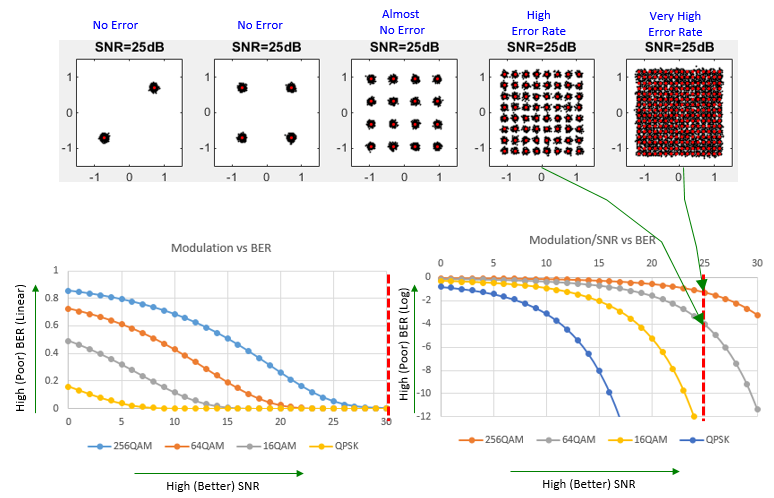Now let's decrease SNR by another 5 dB. On the top track, you would notice that the range of errors on constellation gets even more wider and you see the rate of Bit Error increases even more on the plot.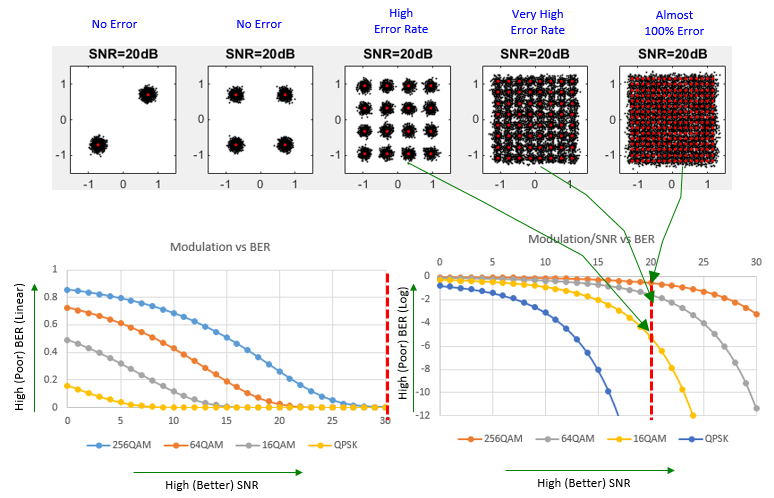Now let's decrease SNR by another 5 dB. On the top track, you would notice that the range of errors on constellation gets even more wider and you see the rate of Bit Error increases even more on the plot.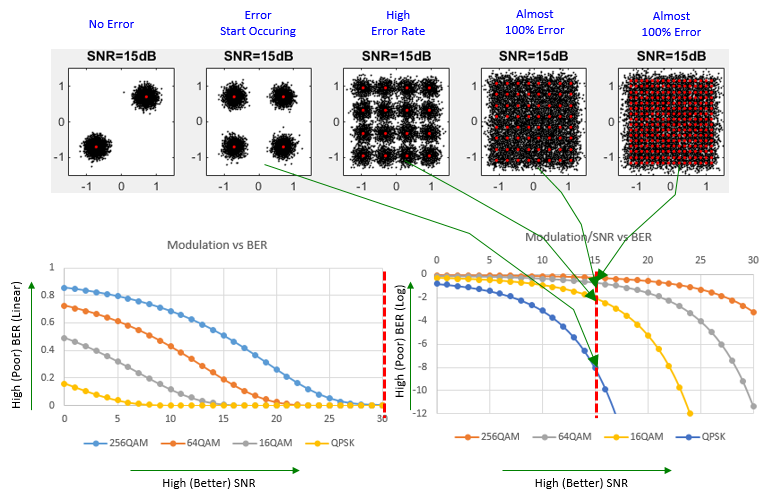Now would you see any trend from this example ?  Even with exactly same constellation, Bit Error Rate increase or decrease based on SNR.  Many people tend to think that the error rate is determined by transmitter power and reciever power, but in reality the absolute power is not important. The thing that is really important is SNR. However, in practice many people including me take transmitter or reciever power as an indirect indicator for SNR based on 'BIG ASSUMPTION' that the level of noise is known (even roughly) and the level of noise does not change when you increase or decrease power. If this BIG ASSUMPTION holds true, if you increase Transmitter power you may say SNR would be better than the case when you have low transmitter power. If you have higher recieved power, you may say SNR would be better than the case when you lower reciever power. But don't blindly apply this rule for any accurate analysis or troubleshooting. If you are in stuation where you need very accurate analysis of Bit Error analysis, you need to check SNR of every components on the signal path. I know this is huge job, this is one of the reason why it take such a long time with using a lot of high end test equipment for calibrating the high accuracy test equipment (e.g, Conformance Test system).   As you see above, you might have noticed that SNR is tightly related to BER (Bit Error Rate). You might have seen a kind of general trend as follows : i) At the same modulation depth, you will get high BER(Poor Performance) at low SNR and low BER (Good Performance) at high SNR ii) At the same SNR, you will get high BER(Poor Performance) at high modulation depth and low BER (Good Performance) at low modulation depth However, in modern communication various kinds of channel coding and error correction technology is used to correct the certain degree of BER. So if you measure the error rate after error correction, you may see much lower error rate than the case without error correction. Usually the error rate after the error correction is measured as a parameter called BLER (BLock Error Rate). However, even with this kind of error correction process, you cannot fix all the errors. Therefore, the general trend still holds true at BLER measurement. i) At the same modulation depth, you will get high BLER(Poor Performance) at low SNR and low BLER (Good Performance) at high SNR ii) At the same SNR, you will get high BLER(Poor Performance) at high modulation depth and low BLER (Good Performance) at low modulation depth The exact correlation between SNR and BLER may vary depending on what kind of channel coding and error correction are used. Following graph shows a good example of SNR vs BLER for LTE PDSCH (See Ref  for the details. this is data for the system supporting only up to 64 QAM. You would see different plots if you measure with the system supporting 256 QAM).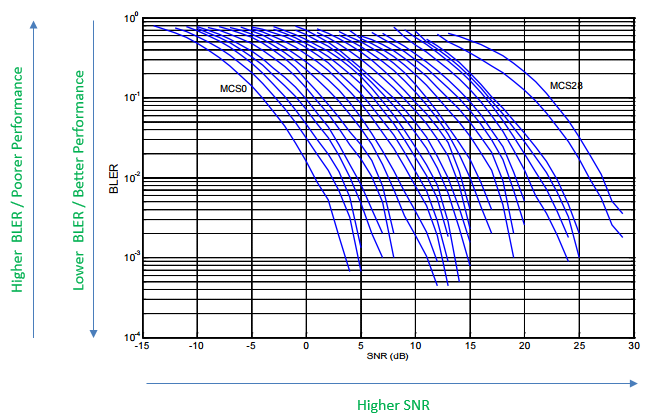SINAD (Signal to Noise And Distortion Ratio)   Similar to SNR, there is another indicator called SINAD. It is defined as shown below. It indicates the ratio of Total energy (Wanted + Unwanted) and Unwanted power. Since the numerator is the total power in the definition, the value in dB is always positive.In most of RF area, we use SNR more frequently and in some area like Audio signal analysis we tend to use SINAD more frequently.   We often get confused by SNR vs SINAD and have difficulties in understanding the difference between SNR and SINAD. It is well explained in Reference  as stated below.   Signal-to-noise ratio (SNR, or sometimes called SNR-without-harmonics) is calculated from the FFT data the same as SINAD, except that the signal harmonics are excluded from the calculation, leaving only the noise terms. In practice, it is only necessary to exclude the first 5 harmonics, since they dominate. The SNR plot will degrade at high input frequencies, but generally not as rapidly as SINAD because of the exclusion of the harmonic terms.   As stated above, the main difference is whether to include 'distortion' in the calculation or not. Distortion can be more intuitively understood in time domain. If you convert the signal with distortion into frequency domain, the distortion appears in the form of harmonics. So in terms of frequency domain, the main difference between SNR and SINAD is whether to include harmonics in the calculation or not.       SINR (Signal to Interference plus Noise Ratio)   SINR stands for Signal to Interference plus Noise Ratio and the definition can be illustrated as below (I hope this single picture can explain everything). Simply put, SINR is the ratio of the signal (desired signal) and the unwanted noise. The unwanted noise comprises of all the external interference and internaly generated noise.Example 1 : SNR (SINR) vs Throughput in a LTE Live Network   Following plot is from the data captured by a drive test tool Azenqos Drive Test tool (AZQ Android). This plot is automatically generated by AZQ Reporting tool and I just did some cosmetic touch on the chart. This is the real measurement showing the correlation between SINR and Throughput. As you see, as SNR(SINR) goes higher, throughput increases exponentially. In other words, As SNR decreases, the throughput will decrease exponetially. If network does not change code rate (i.e, MCS), the throughput decrease would be due to decoding failure at the reciever (i.e, decoding failure at UE), however in real network UE reports CQI periodically to eNB and eNB changes the code rate accordinly (i.e, decreasing MCS as CQI value gets lower and this results in smaller transport block size), so this throughput change would be due to lower transport block size.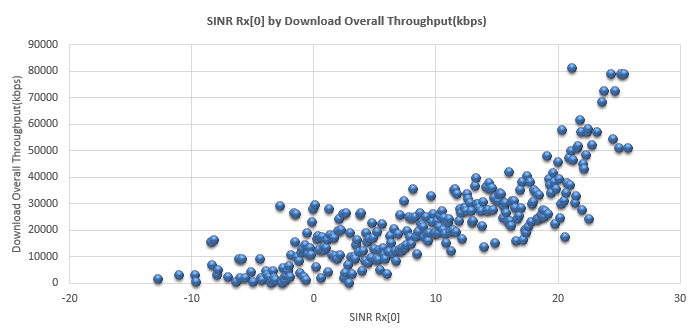Reference :    NISTIR 7986 - LTE Physical Layer Performance Analysis by Wen-Bin Yang, Michael Souryal